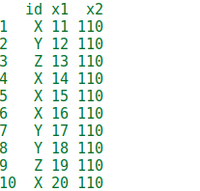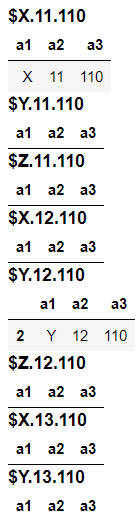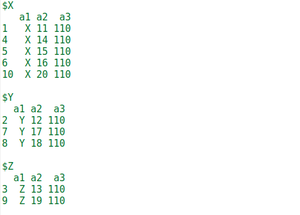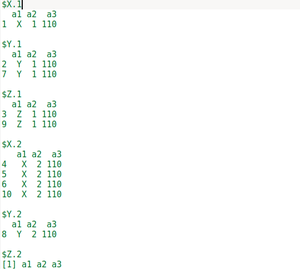Open in App
Not now

# Split large R Dataframe into list of smaller Dataframes

• Last Updated : 06 Aug, 2021

In this article, we will discuss how to split a large R dataframe into lists of smaller Dataframes. In R Programming language we have a function named split() which is used to split the data frame into parts.

So to do this, we first create an example of a dataframe which is needed to be split.

Creating dataframe:

## R

 `# create the data frame` `data <- ``data.frame``(id = ``c``(``"X"``, ``"Y"``, ``"Z"``, ``"X"``, ``"X"``,` `                          ``"X"``, ``"Y"``, ``"Y"``, ``"Z"``, ``"X"``),  ` `                   ``x1 = 11 : 20,` `                   ``x2 = 110 : 110)`   `# print the dataframe` `data`

Output:To split the above Dataframe we use the split() function. The syntax of split() function is:

Syntax: split(x, f, drop = FALSE, …)

Parameters:

• x stands for DataFrame and vector
• f stands for grouping of vector or selecting the column according to which we split the Dataframe
• drop stands for delete or skip the specified row

Example 1: In this example, we try to run the split function without any argument except the above Dataframe.

When we run the split function without any argument except dataframe we noticed that the split function returns the combination of every element of column 1 with the other columns. In our case, there are 3 distinct elements in column 1 and a total of 10 rows in the data frame. So, the total rows as output are 3 * 10 = 30 rows in our output.

## R

 `# create the data frame` `data <- ``data.frame``(a1 = ``c``(``"X"``, ``"Y"``, ``"Z"``, ``"X"``, ``"X"``,` `                          ``"X"``, ``"Y"``, ``"Y"``, ``"Z"``, ``"X"``),  ` `                   ``a2 = 11 : 20,` `                   ``a3 = 110 : 110)`   `# split the dataframe using the` `# split function` `split_data <- ``split``(data, f = data)    `   `# print the splitted data frame` `split_data`

Output:Note: The above output screenshot is 1/3 of the actual output, due to conciseness we can not insert the full output screenshot.

Example 2: In this example, we will split the Dataframe by grouping with the help of 1 column.

To do this we will use the “f” argument of the split function and “\$” is used to selecting the column according to which we are going to split the Dataframe. In our case, we are going to split the Dataframe according to the a1 column.

## R

 `# create the data frame` `data <- ``data.frame``(a1 = ``c``(``"X"``, ``"Y"``, ``"Z"``, ``"X"``, ``"X"``,` `                          ``"X"``, ``"Y"``, ``"Y"``, ``"Z"``, ``"X"``),  ` `                   ``a2 = 11 : 20,` `                   ``a3 = 110 : 110)`   `# split the data frame by grouping using "f" argument` `split_data <- ``split``(data, f = data\$a1)   `   `# print the split data` `split_data`

Output:Example 3: In this example, we will split the Dataframe by grouping with the help of 2 columns.

To do this we will use the “f” argument of the split function and “\$” is used to selecting the columns and make a list of the columns according to which we are going to split the Dataframe. In our case, we are going to split the Dataframe according to the a1 and a2 columns. So, a list of a1 and a2 is created and this list is given as an argument to the “f”.

## R

 `# create the data frame` `data <- ``data.frame``(a1 = ``c``(``"X"``, ``"Y"``, ``"Z"``, ``"X"``, ``"X"``,` `                          ``"X"``, ``"Y"``, ``"Y"``, ``"Z"``, ``"X"``),  ` `                   `  `                   ``a2 = ``c``(1, 1, 1, 2, 2, 2,` `                          ``1, 2, 1, 2),` `                   ``a3 = 110 : 110)` `# split the data frame by grouping using "f" argument` `split_data <- ``split``(data, f=``list``(data\$a1, data\$a2))   `   `# print the split data` `split_data`

Output:My Personal Notes arrow_drop_up
Related Articles